# Cuckoo Hashing – Worst case O(1) Lookup!

• Difficulty Level : Hard
• Last Updated : 01 Jul, 2022

Background :

There are three basic operations that must be supported by a hash table (or a dictionary):

• Lookup(key): return true if key is there on the table, else false
• Insert(key): add the item ‘key’ to the table if not already present
• Delete(key): removes ‘key’ from the table

Collisions are very likely even if we have a big table to store keys. Using the results from the birthday paradox: with only 23 persons, the probability that two people share the same birth date is 50%! There are 3 general strategies towards resolving hash collisions:

• Closed addressing or Chaining: store colliding elements in an auxiliary data structure like a linked list or a binary search tree.
• Open addressing: allow elements to overflow out of their target bucket and into other spaces.

Although above solutions provide expected lookup cost as O(1), the expected worst-case cost of a lookup in Open Addressing (with linear probing) is Ω(log n) and Θ(log n / log log n) in simple chaining (Source : Standford Lecture Notes). To close the gap of expected time and worst case expected time, two ideas are used:

• Multiple-choice hashing: Give each element multiple choices for positions where it can reside in the hash table
• Relocation hashing: Allow elements in the hash table to move after being placed

Cuckoo Hashing :

Cuckoo hashing applies the idea of multiple-choice and relocation together and guarantees O(1) worst case lookup time!

• Multiple-choice: We give a key two choices the h1(key) and h2(key) for residing.
• Relocation: It may happen that h1(key) and h2(key) are preoccupied. This is resolved by imitating the Cuckoo bird: it pushes the other eggs or young out of the nest when it hatches. Analogously, inserting a new key into a cuckoo hashing table may push an older key to a different location. This leaves us with the problem of re-placing the older key.
• If the alternate position of older key is vacant, there is no problem.
• Otherwise, the older key displaces another key. This continues until the procedure finds a vacant position, or enters a cycle. In the case of a cycle, new hash functions are chosen and the whole data structure is ‘rehashed’. Multiple rehashes might be necessary before Cuckoo succeeds.

Insertion is expected O(1) (amortized) with high probability, even considering the possibility of rehashing, as long as the number of keys is kept below half of the capacity of the hash table, i.e., the load factor is below 50%.

Deletion is O(1) worst-case as it requires inspection of just two locations in the hash table.

Illustration

Input:

`{20, 50, 53, 75, 100, 67, 105, 3, 36, 39}`

Hash Functions:

```h1(key) = key%11
h2(key) = (key/11)%11```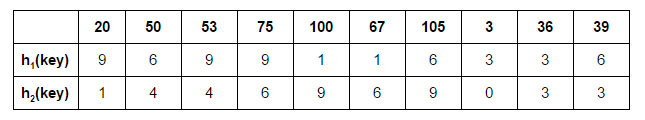Let’s start by inserting 20 at its possible position in the first table determined by h1(20):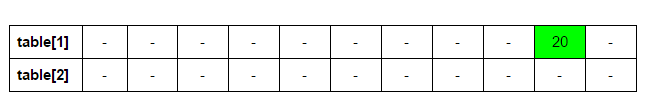Next: 50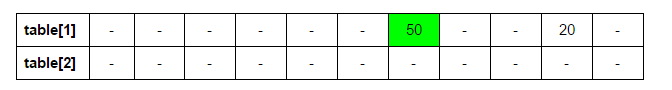Next: 53. h1(53) = 9. But 20 is already there at 9. We place 53 in table 1 & 20 in table 2 at h2(20)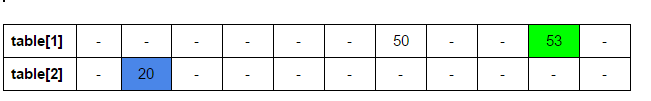Next: 75. h1(75) = 9. But 53 is already there at 9. We place 75 in table 1 & 53 in table 2 at h2(53)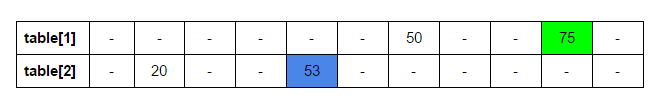Next: 100. h1(100) = 1.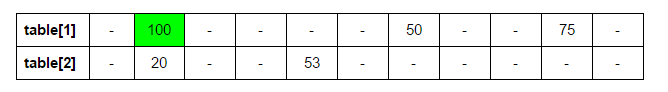Next: 67. h1(67) = 1. But 100 is already there at 1. We place 67 in table 1 & 100 in table 2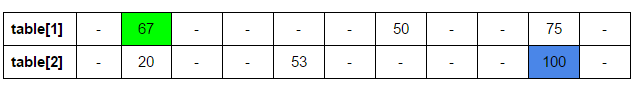Next: 105. h1(105) = 6. But 50 is already there at 6. We place 105 in table 1 & 50 in table 2 at h2(50) = 4. Now 53 has been displaced. h1(53) = 9. 75 displaced: h2(75) = 6.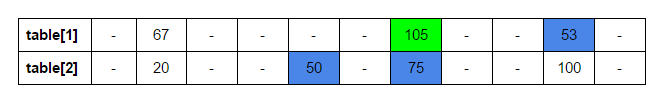Next: 3. h1(3) = 3.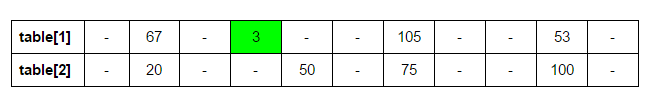Next: 36. h1(36) = 3. h2(3) = 0.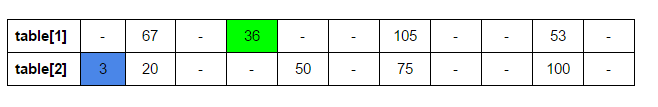Next: 39. h1(39) = 6. h2(105) = 9. h1(100) = 1. h2(67) = 6. h1(75) = 9. h2(53) = 4. h1(50) = 6. h2(39) = 3.
Here, the new key 39 is displaced later in the recursive calls to place 105, which it displaced.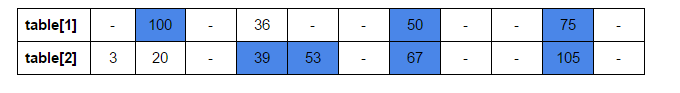Implementation:

Below is the implementation of Cuckoo hashing

## C++

 `// C++ program to demonstrate working of Cuckoo` `// hashing.` `#include`   `// upper bound on number of elements in our set` `#define MAXN 11`   `// choices for position` `#define ver 2`   `// Auxiliary space bounded by a small multiple` `// of MAXN, minimizing wastage` `int` `hashtable[ver][MAXN];`   `// Array to store possible positions for a key` `int` `pos[ver];`   `/* function to fill hash table with dummy value` ` ``* dummy value: INT_MIN` ` ``* number of hashtables: ver */` `void` `initTable()` `{` `    ``for` `(``int` `j=0; j

## Java

 `// Java program to demonstrate working of ` `// Cuckoo hashing.` `import` `java.util.*;`   `class` `GFG ` `{`   `// upper bound on number of elements in our set` `static` `int` `MAXN = ``11``;`   `// choices for position` `static` `int` `ver = ``2``;`   `// Auxiliary space bounded by a small multiple` `// of MAXN, minimizing wastage` `static` `int` `[][]hashtable = ``new` `int``[ver][MAXN];`   `// Array to store possible positions for a key` `static` `int` `[]pos = ``new` `int``[ver];`   `/* function to fill hash table with dummy value` `* dummy value: INT_MIN` `* number of hashtables: ver */` `static` `void` `initTable()` `{` `    ``for` `(``int` `j = ``0``; j < MAXN; j++)` `        ``for` `(``int` `i = ``0``; i < ver; i++)` `            ``hashtable[i][j] = Integer.MIN_VALUE;` `}`   `/* return hashed value for a key` `* function: ID of hash function according to which` `    ``key has to hashed` `* key: item to be hashed */` `static` `int` `hash(``int` `function, ``int` `key)` `{` `    ``switch` `(function)` `    ``{` `        ``case` `1``: ``return` `key % MAXN;` `        ``case` `2``: ``return` `(key / MAXN) % MAXN;` `    ``}` `    ``return` `Integer.MIN_VALUE;` `}`   `/* function to place a key in one of its possible positions` `* tableID: table in which key has to be placed, also equal` `  ``to function according to which key must be hashed` `* cnt: number of times function has already been called` `  ``in order to place the first input key` `* n: maximum number of times function can be recursively` `  ``called before stopping and declaring presence of cycle */` `static` `void` `place(``int` `key, ``int` `tableID, ``int` `cnt, ``int` `n)` `{` `    ``/* if function has been recursively called max number` `    ``of times, stop and declare cycle. Rehash. */` `    ``if` `(cnt == n)` `    ``{` `        ``System.out.printf(``"%d unpositioned\n"``, key);` `        ``System.out.printf(``"Cycle present. REHASH.\n"``);` `        ``return``;` `    ``}`   `    ``/* calculate and store possible positions for the key.` `    ``* check if key already present at any of the positions.` `    ``If YES, return. */` `    ``for` `(``int` `i = ``0``; i < ver; i++)` `    ``{` `        ``pos[i] = hash(i + ``1``, key);` `        ``if` `(hashtable[i][pos[i]] == key)` `        ``return``;` `    ``}`   `    ``/* check if another key is already present at the` `       ``position for the new key in the table` `    ``* If YES: place the new key in its position` `    ``* and place the older key in an alternate position` `    ``for it in the next table */` `    ``if` `(hashtable[tableID][pos[tableID]] != Integer.MIN_VALUE)` `    ``{` `        ``int` `dis = hashtable[tableID][pos[tableID]];` `        ``hashtable[tableID][pos[tableID]] = key;` `        ``place(dis, (tableID + ``1``) % ver, cnt + ``1``, n);` `    ``}` `    ``else` `// else: place the new key in its position` `    ``hashtable[tableID][pos[tableID]] = key;` `}`   `/* function to print hash table contents */` `static` `void` `printTable()` `{` `    ``System.out.printf(``"Final hash tables:\n"``);`   `    ``for` `(``int` `i = ``0``; i < ver; i++, System.out.printf(``"\n"``))` `        ``for` `(``int` `j = ``0``; j < MAXN; j++)` `            ``if``(hashtable[i][j] == Integer.MIN_VALUE) ` `                ``System.out.printf(``"- "``);` `            ``else` `                ``System.out.printf(``"%d "``, hashtable[i][j]);`   `    ``System.out.printf(``"\n"``);` `}`   `/* function for Cuckoo-hashing keys` `* keys[]: input array of keys` `* n: size of input array */` `static` `void` `cuckoo(``int` `keys[], ``int` `n)` `{` `    ``// initialize hash tables to a dummy value ` `    ``// (INT-MIN) indicating empty position` `    ``initTable();`   `    ``// start with placing every key at its position in` `    ``// the first hash table according to first hash` `    ``// function` `    ``for` `(``int` `i = ``0``, cnt = ``0``; i < n; i++, cnt = ``0``)` `        ``place(keys[i], ``0``, cnt, n);`   `    ``// print the final hash tables` `    ``printTable();` `}`   `// Driver Code` `public` `static` `void` `main(String[] args) ` `{` `    ``/* following array doesn't have any cycles and` `    ``hence all keys will be inserted without any` `    ``rehashing */` `    ``int` `keys_1[] = {``20``, ``50``, ``53``, ``75``, ``100``, ` `                    ``67``, ``105``, ``3``, ``36``, ``39``};`   `    ``int` `n = keys_1.length;`   `    ``cuckoo(keys_1, n);`   `    ``/* following array has a cycle and hence we will` `    ``have to rehash to position every key */` `    ``int` `keys_2[] = {``20``, ``50``, ``53``, ``75``, ``100``, ` `                    ``67``, ``105``, ``3``, ``36``, ``39``, ``6``};`   `    ``int` `m = keys_2.length;`   `    ``cuckoo(keys_2, m);` `}` `} `   `// This code is contributed by Princi Singh`

## C#

 `// C# program to demonstrate working of ` `// Cuckoo hashing.` `using` `System;` `    `  `class` `GFG ` `{`   `// upper bound on number of` `// elements in our set` `static` `int` `MAXN = 11;`   `// choices for position` `static` `int` `ver = 2;`   `// Auxiliary space bounded by a small ` `// multiple of MAXN, minimizing wastage` `static` `int` `[,]hashtable = ``new` `int``[ver, MAXN];`   `// Array to store ` `// possible positions for a key` `static` `int` `[]pos = ``new` `int``[ver];`   `/* function to fill hash table ` `with dummy value` `* dummy value: INT_MIN` `* number of hashtables: ver */` `static` `void` `initTable()` `{` `    ``for` `(``int` `j = 0; j < MAXN; j++)` `        ``for` `(``int` `i = 0; i < ver; i++)` `            ``hashtable[i, j] = ``int``.MinValue;` `}`   `/* return hashed value for a key` `* function: ID of hash function ` `according to which key has to hashed` `* key: item to be hashed */` `static` `int` `hash(``int` `function, ``int` `key)` `{` `    ``switch` `(function)` `    ``{` `        ``case` `1: ``return` `key % MAXN;` `        ``case` `2: ``return` `(key / MAXN) % MAXN;` `    ``}` `    ``return` `int``.MinValue;` `}`   `/* function to place a key in one of ` `its possible positions` `* tableID: table in which key ` `has to be placed, also equal to function` `according to which key must be hashed` `* cnt: number of times function has already ` `been called in order to place the first input key` `* n: maximum number of times function ` `can be recursively called before stopping and` `declaring presence of cycle */` `static` `void` `place(``int` `key, ``int` `tableID, ` `                  ``int` `cnt, ``int` `n)` `{` `    ``/* if function has been recursively ` `    ``called max number of times,` `    ``stop and declare cycle. Rehash. */` `    ``if` `(cnt == n)` `    ``{` `        ``Console.Write(``"{0} unpositioned\n"``, key);` `        ``Console.Write(``"Cycle present. REHASH.\n"``);` `        ``return``;` `    ``}`   `    ``/* calculate and store possible positions ` `    ``* for the key. Check if key already present ` `    ``at any of the positions. If YES, return. */` `    ``for` `(``int` `i = 0; i < ver; i++)` `    ``{` `        ``pos[i] = hash(i + 1, key);` `        ``if` `(hashtable[i, pos[i]] == key)` `        ``return``;` `    ``}`   `    ``/* check if another key is already present ` `    ``at the position for the new key in the table` `    ``* If YES: place the new key in its position` `    ``* and place the older key in an alternate position` `    ``for it in the next table */` `    ``if` `(hashtable[tableID, ` `              ``pos[tableID]] != ``int``.MinValue)` `    ``{` `        ``int` `dis = hashtable[tableID, pos[tableID]];` `        ``hashtable[tableID, pos[tableID]] = key;` `        ``place(dis, (tableID + 1) % ver, cnt + 1, n);` `    ``}` `    ``else` `// else: place the new key in its position` `    ``hashtable[tableID, pos[tableID]] = key;` `}`   `/* function to print hash table contents */` `static` `void` `printTable()` `{` `    ``Console.Write(``"Final hash tables:\n"``);`   `    ``for` `(``int` `i = 0; i < ver;` `             ``i++, Console.Write(``"\n"``))` `        ``for` `(``int` `j = 0; j < MAXN; j++)` `            ``if``(hashtable[i, j] == ``int``.MinValue) ` `                ``Console.Write(``"- "``);` `            ``else` `                ``Console.Write(``"{0} "``,` `                        ``hashtable[i, j]);`   `    ``Console.Write(``"\n"``);` `}`   `/* function for Cuckoo-hashing keys` `* keys[]: input array of keys` `* n: size of input array */` `static` `void` `cuckoo(``int` `[]keys, ``int` `n)` `{` `    ``// initialize hash tables to a ` `    ``// dummy value (INT-MIN)` `    ``// indicating empty position` `    ``initTable();`   `    ``// start with placing every key ` `    ``// at its position in the first ` `    ``// hash table according to first ` `    ``// hash function` `    ``for` `(``int` `i = 0, cnt = 0;` `             ``i < n; i++, cnt = 0)` `        ``place(keys[i], 0, cnt, n);`   `    ``// print the final hash tables` `    ``printTable();` `}`   `// Driver Code` `public` `static` `void` `Main(String[] args) ` `{` `    ``/* following array doesn't have ` `    ``any cycles and hence all keys ` `    ``will be inserted without any rehashing */` `    ``int` `[]keys_1 = {20, 50, 53, 75, 100, ` `                    ``67, 105, 3, 36, 39};`   `    ``int` `n = keys_1.Length;`   `    ``cuckoo(keys_1, n);`   `    ``/* following array has a cycle and ` `    ``hence we will have to rehash to ` `    ``position every key */` `    ``int` `[]keys_2 = {20, 50, 53, 75, 100, ` `                    ``67, 105, 3, 36, 39, 6};`   `    ``int` `m = keys_2.Length;`   `    ``cuckoo(keys_2, m);` `}` `}`   `// This code is contributed by PrinciRaj1992`

## Javascript

 ``

Output

```Final hash tables:
- 100 - 36 - - 50 - - 75 -
3 20 - 39 53 - 67 - - 105 -

105 unpositioned
Cycle present. REHASH.
Final hash tables:
- 67 - 3 - - 39 - - 53 -
6 20 - 36 50 - 75 - - 100 -

```

Generalizations of cuckoo hashing that use more than 2 alternative hash functions can be expected to utilize a larger part of the capacity of the hash table efficiently while sacrificing some lookup and insertion speed. Example: if we use 3 hash functions, it’s safe to load 91% and still be operating within expected bounds.

This article is contributed by Yash Varyani. If you like GeeksforGeeks and would like to contribute, you can also write an article and mail your article to review-team@geeksforgeeks.org. See your article appearing on the GeeksforGeeks main page and help other Geeks.

My Personal Notes arrow_drop_up
Recommended Articles
Page :